#### How to Calculate WACC in Excel

WACC, which stands for Weighted Average Cost of Capital, is the way a company can measure how much its capital costs. The WACC indicator is expressed as a percentage and is most often used to assess the attractiveness of an investment.

It is crucial to have some kind of data before calculating the Weighted Average Cost of Capital.

## Data preparation

List the various sources of capital for the company, such as equity, debt, preferred stock, etc. This data would looks something like this: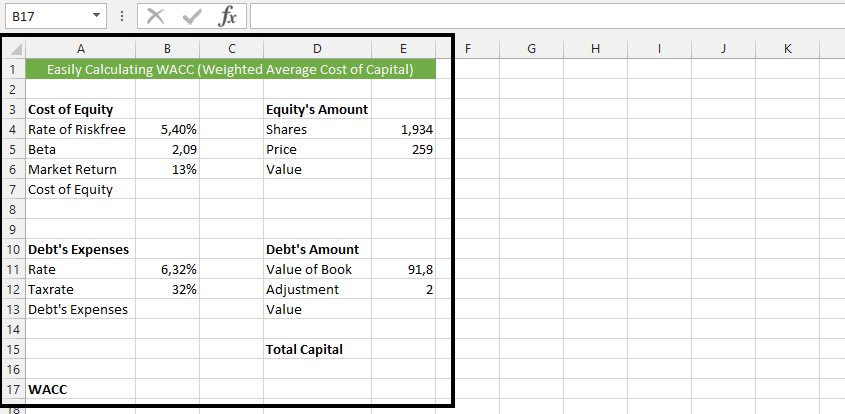## Wacc calculations

To calculate the cost of equity, click on B7 (1), type =B4+B5*(B6-B4) (2), and press enter.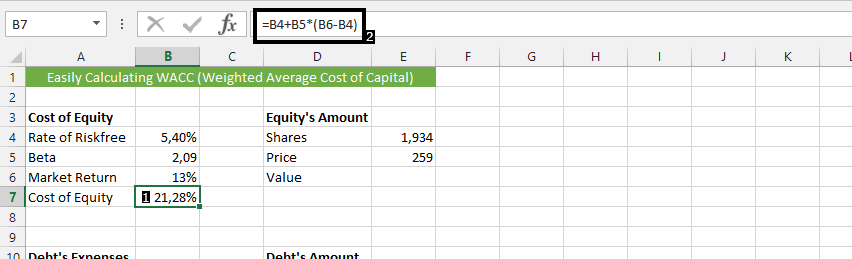If the result is a number, use the format cell to convert it to a percentage.

Click on the cell beside the debt’s expenses (1), and type in =B11*(1-B12) (2), and then press enter.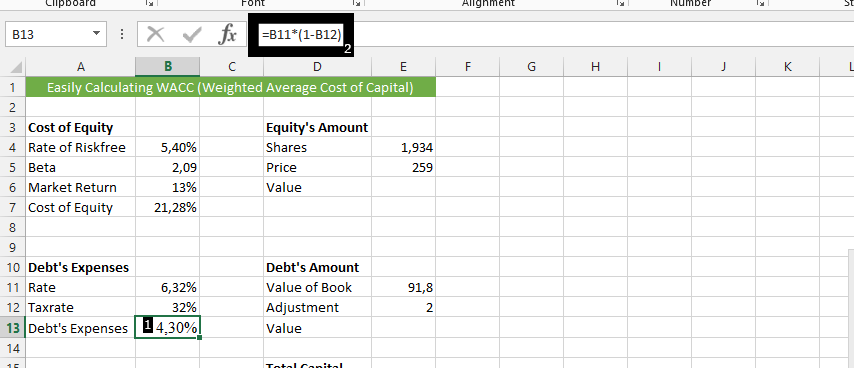Click on the cell beside Value (1), and type =E4*E5 (2), to calculate the value on Equity’s amount.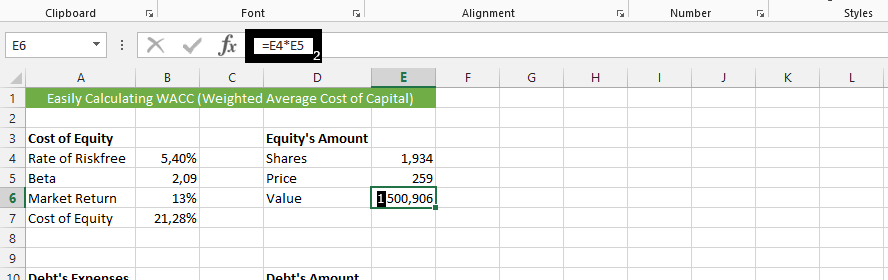Click on beside the value under the debt’s amount (1), and type in =E11*E12, and then enter.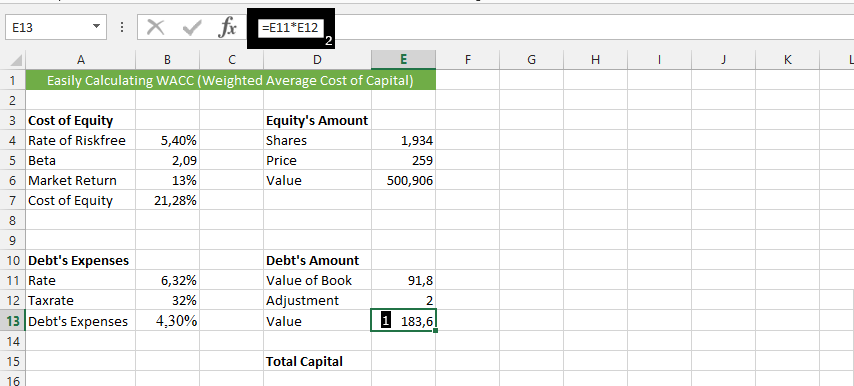Click beside Total capital (1) and type in =E6+E13 (2).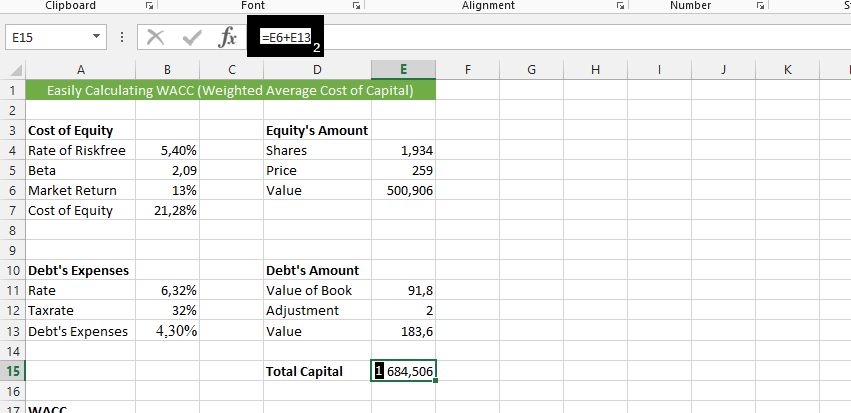Click the cell next to WACC (1), enter =B7*E6/E15+B13*E13/E15, and press enter.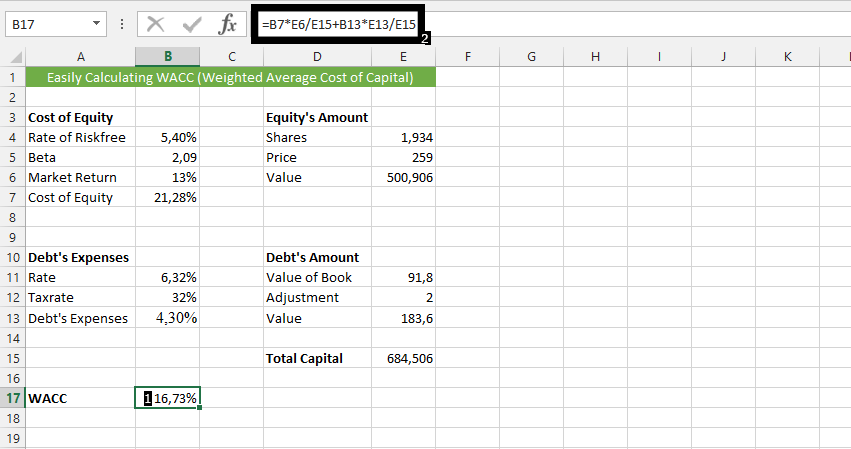This is how to calculate WACC in Excel. The formula for WACC in Excel is: = SUMPRODUCT(Cost * Weight) / SUM(Weight). You can use this as well.

By using this formula in Excel, you can easily calculate the WACC for a company, which provides important insight into the cost of capital for the company and its ability to generate returns for its shareholders.

## Wacc calculations using weighted average method

Here’s an example of how to calculate WACC using a simpler approach, known as the weighted average method:

1. Determine the cost of each source of capital:

To calculate WACC, you’ll need to know the cost of each source of capital. This includes the cost of debt and the cost of equity. Let’s assume that the cost of debt is 6% and the cost of equity is 12%.

1. Determine the market value of each source of capital:

Next, you’ll need to determine the market value of each source of capital. This includes the market value of debt and the market value of equity. For this example, let’s assume that the market value of debt is \$2 million and the market value of equity is \$4 million.

1. Calculate the weighted average cost of capital:

To calculate the WACC using the weighted average method, follow these steps:

• Determine the total market value of the company’s capital by adding the market value of debt and the market value of equity: \$2 million + \$4 million = \$6 million.
• Determine the market value weight of each source of capital by dividing the market value of each source of capital by the total market value of the company’s capital:
• Market value weight of debt: \$2 million / \$6 million = 0.33
• Market value weight of equity: \$4 million / \$6 million = 0.67
• Multiply the cost of debt by the market value weight of debt and the cost of equity by the market value weight of equity:
• Weighted cost of debt: 6% x 0.33 = 1.98%
• Weighted cost of equity: 12% x 0.67 = 8.04%
• Add the weighted cost of debt and the weighted cost of equity to get the WACC:
• WACC: 1.98% + 8.04% = 10.02%

Therefore, the WACC for this company using the weighted average method is 10.02%.

Note that this method is simpler but assumes that the company has a fixed amount of debt and equity, and that their market values and costs do not change over time. This is usually not the case for real-world companies, so the WACC calculated using this method may not be as accurate as the SUMPRODUCT method that takes into account the changing market values and costs of each source of capital.

## Wacc calculations using RATE function

The RATE function is a built-in Excel function that can be used to calculate the WACC by iteratively solving for the discount rate that equates the present value of the company’s cash flows to the total market value of the company’s capital. Here’s an example of how to use the RATE function:

1. Determine the market value of each source of capital:

To use the RATE function to calculate WACC, you’ll need to know the market value of each source of capital. Let’s assume that the market value of debt is \$2 million and the market value of equity is \$4 million.

1. Determine the cost of each source of capital:

Next, you’ll need to determine the cost of each source of capital. This includes the cost of debt and the cost of equity. For this example, let’s assume that the cost of debt is 6% and the cost of equity is 12%.

1. Determine the present value of the company’s cash flows:

The present value of the company’s cash flows is the sum of the present value of the company’s debt and the present value of the company’s equity. To calculate this value, you’ll need to know the expected cash flows for each source of capital and the discount rate.

• Present value of debt: To calculate the present value of the company’s debt, you’ll need to know the expected cash flows from the debt and the discount rate. Let’s assume that the expected cash flows from the debt are \$200,000 per year for 10 years. The present value of this stream of cash flows at a discount rate of 6% can be calculated using the Excel PV function: =PV(6%, 10, 200000) = \$1,486,852.
• Present value of equity: To calculate the present value of the company’s equity, you’ll need to know the expected cash flows from the equity and the discount rate. Let’s assume that the expected cash flows from the equity are \$400,000 per year indefinitely. The present value of this stream of cash flows at a discount rate of 12% can be calculated using the Excel PV function: =PV(12%, 0, 400000) / 0.12 = \$3,333,333.
• Total present value of cash flows: Add the present value of the company’s debt and the present value of the company’s equity to get the total present value of the company’s cash flows: \$1,486,852 + \$3,333,333 = \$4,820,185.
1. Use the RATE function to calculate WACC:

The RATE function can be used to solve for the discount rate that equates the present value of the company’s cash flows to the total market value of the company’s capital. In this case, the total market value of the company’s capital is \$2 million + \$4 million = \$6 million. The syntax for the RATE function is:

=RATE(nper, pmt, pv, [fv], [type], [guess])

Where:

• nper is the total number of periods
• pmt is the payment made each period
• pv is the present value of the cash flows
• fv is the future value of the cash flows (optional)
• type is the type of payment made each period (optional)
• guess is the initial guess for the discount rate (optional)

In this case, nper is 10, pmt is -\$200,000 (the expected cash flow from the debt), and pv is -\$4,820,185 (the total present value of the company’s cash flows). The

full formula using the RATE function is:

=RATE(10, -200000, -4820185, , , )

When you enter this formula into Excel, it will return the WACC for the company, which is 8.48%.

Note that the RATE function is an iterative function, which means that it solves for the discount rate by making a series of guesses and adjusting the discount rate until it finds the correct value. The function may take several seconds or even minutes to complete, depending on the complexity of the calculation and the number of iterations required to find the correct value.

In addition, the RATE function assumes that the cash flows are evenly distributed over the life of the investment. If the cash flows are not evenly distributed, you may need to use a different formula or a different method to calculate the WACC.

Overall, the RATE function is a powerful tool that can be used to calculate the WACC in Excel. With a good understanding of the inputs and outputs of the function, you can use this tool to make informed decisions about the cost of capital for your business or investment.

## Key Takeaways

• WACC, which stands for Weighted Average Cost of Capital, is the way a company can measure how much its capital costs.
• There are two main methods for calculating WACC: the weighted average method and the RATE function.
• The weighted average method is simpler but assumes that the company has a fixed amount of debt and equity, and that their market values and costs do not change over time.
• The RATE function is a more complex method but takes into account the changing market values and costs of each source of capital.

## FAQ

• Q: What is the weighted average method?
• A: The weighted average method is a method for calculating WACC that takes into account the market value of each source of capital and the cost of each source of capital.
• Q: What is the RATE function?
• A: The RATE function is a built-in Excel function that can be used to calculate WACC by iteratively solving for the discount rate that equates the present value of the company’s cash flows to the total market value of the company’s capital.
• Q: What are the benefits of using the RATE function?
• A: The RATE function has several benefits, including that it is more accurate than the weighted average method and that it can be used to calculate WACC for companies with non-evenly distributed cash flows.